UWP开发随笔——使用SQLite数据库

0
0
01. 云栖社区>
2. 博客>
3. 正文

摘要

环境配置

1.下载和安装SQLite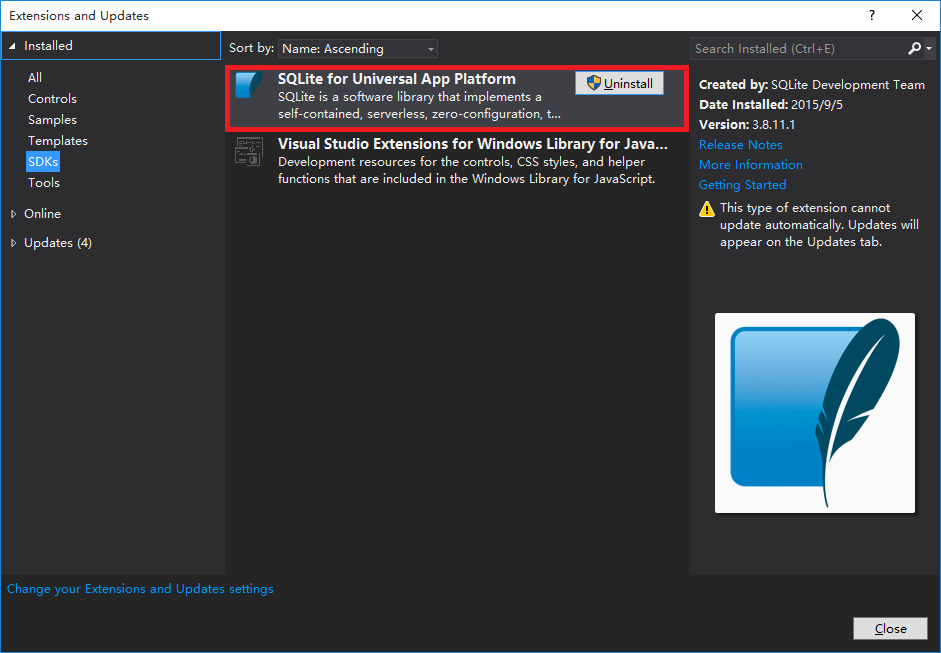2.在工程中添加对SQLite的引用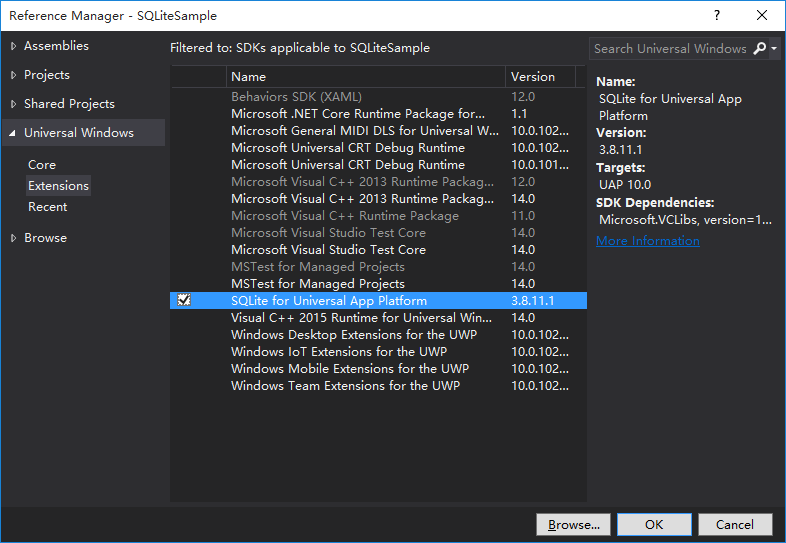3.添加.Net支持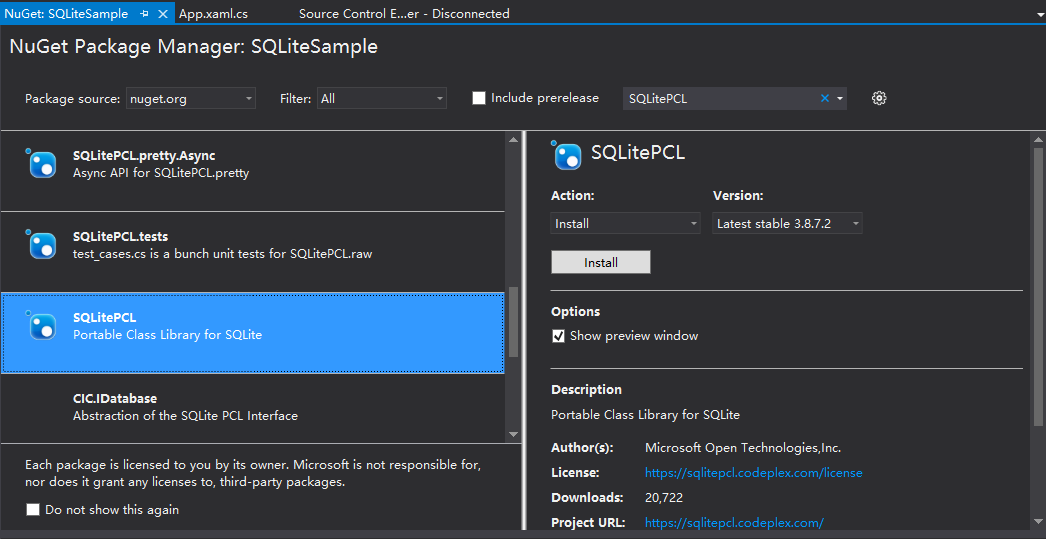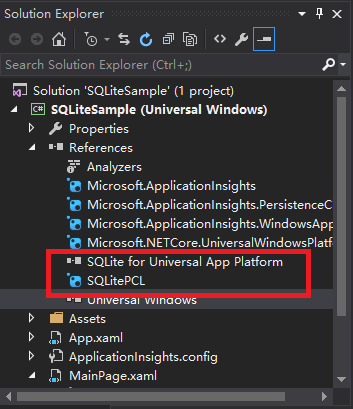定义SQL语句常量

private static String DB_NAME = "SQLiteSample.db";
private static String TABLE_NAME = "SampleTable";
private static String SQL_CREATE_TABLE = "CREATE TABLE IF NOT EXISTS " + TABLE_NAME + " (Key TEXT,Value TEXT);";
private static String SQL_QUERY_VALUE = "SELECT Value FROM " + TABLE_NAME + " WHERE Key = (?);";
private static String SQL_INSERT = "INSERT INTO " + TABLE_NAME + " VALUES(?,?);";
private static String SQL_UPDATE = "UPDATE " + TABLE_NAME + " SET Value = ? WHERE Key = ?";
private static String SQL_DELETE = "DELETE FROM " + TABLE_NAME + " WHERE Key = ?"

建立第一张表

_connection = new SQLiteConnection(DB_NAME);
using (var statement = _connection.Prepare(SQL_CREATE_TABLE))
{
statement.Step();
}

INSERT

using (var statement = _connection.Prepare(SQL_INSERT))
{
statement.Bind(1, key);
statement.Bind(2, value);
statement.Step();
}

DELETE

using (var statement = _connection.Prepare(SQL_DELETE))
{
statement.Bind(1, key);
statement.Step();
}

UPDATE

using(var statement = _connection.Prepare(SQL_UPDATE))
{
statement.Bind(1, value);
statement.Bind(2, key);
statement.Step();
}

QUERY

using (var statement = _connection.Prepare(SQL_QUERY_VALUE))
{
statement.Bind(1, key);
SQLiteResult result = statement.Step();
if (SQLiteResult.ROW == result)
{
value = statement as String;
}
}

其它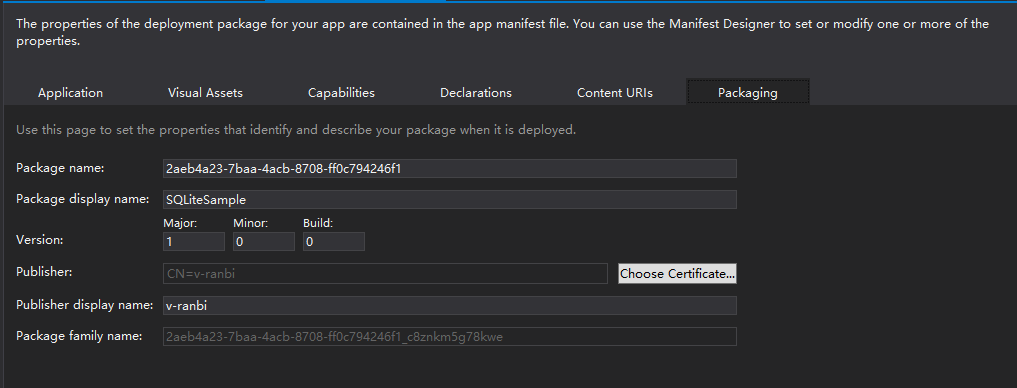+ 关注